# M/J Accelerated Mathematics Grade 7 (#1205050)

This document was generated on CPALMS - www.cpalms.org
You are not viewing the current course, please click the current year’s tab.

#### Course Standards

Name Description
MA.7.AR.2.2: Write and solve two-step equations in one variable within a mathematical or real-world context, where all terms are rational numbers.
 Clarifications:Clarification 1: Instruction focuses the application of the properties of equality. Refer to Properties of Operations, Equality and Inequality (Appendix D). Clarification 2: Instruction includes equations in the forms px±q=r and p(x±q)=r, where p, q and r are specific rational numbers. Clarification 3: Problems include linear equations where the variable may be on either side of the equal sign.
MA.7.AR.3.3: Solve mathematical and real-world problems involving the conversion of units across different measurement systems.
 Examples:Clarification 1: Problem types are limited to length, area, weight, mass, volume and money.
MA.7.AR.4.1: Determine whether two quantities have a proportional relationship by examining a table, graph or written description.
 Clarifications:Clarification 1: Instruction focuses on the connection to ratios and on the constant of proportionality, which is the ratio between two quantities in a proportional relationship.
MA.7.AR.4.2: Determine the constant of proportionality within a mathematical or real-world context given a table, graph or written description of a proportional relationship.
 Examples:Example: A graph has a line that goes through the origin and the point (5,2). This represents a proportional relationship and the constant of proportionality is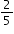. Example: Gina works as a babysitter and earns \$9 per hour. She can only work 6 hours this week. Gina wants to know how much money she will make. Gina can use the equation e=9h, where e is the amount of money earned, h is the number of hours worked and 9 is the constant of proportionality.
MA.7.AR.4.3: Given a mathematical or real-world context, graph proportional relationships from a table, equation or a written description.
 Clarifications:Clarification 1: Instruction includes equations of proportional relationships in the form of y=px, where p is the constant of proportionality.
MA.7.AR.4.4: Given any representation of a proportional relationship, translate the representation to a written description, table or equation.
 Clarifications:Clarification 1: Given representations are limited to a written description, graph, table or equation. Clarification 2: Instruction includes equations of proportional relationships in the form of y=px, where p is the constant of proportionality.

 Examples:Example: The written description, there are 60 minutes in 1 hour, can be represented as the equation m=60h. Example: Gina works as a babysitter and earns \$9 per hour. She would like to earn \$100 to buy a new tennis racket. Gina wants to know how many hours she needs to work. She can use the equation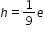, where e is the amount of money earned, h is the number of hours worked and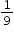is the constant of proportionality.
MA.7.AR.4.5: Solve real-world problems involving proportional relationships.
 Examples:Gordy is taking a trip from Tallahassee, FL to Portland, Maine which is about 1,407 miles. On average his SUV gets 23.1 miles per gallon on the highway and his gas tanks holds 17.5 gallons. If Gordy starts with a full tank of gas, how many times will he be required to fill the gas tank?
MA.7.DP.1.4: Use proportional reasoning to construct, display and interpret data in circle graphs.
 Clarifications:Clarification 1: Data is limited to no more than 6 categories.
MA.7.DP.1.5: Given a real-world numerical or categorical data set, choose and create an appropriate graphical representation.
 Clarifications:Clarification 1: Graphical representations are limited to histograms, bar charts, circle graphs, line plots, box plots and stem-and-leaf plots.
MA.7.GR.1.3: Explore the proportional relationship between circumferences and diameters of circles. Apply a formula for the circumference of a circle to solve mathematical and real-world problems.
 Clarifications:Clarification 1: Instruction includes the exploration and analysis of circular objects to examine the proportional relationship between circumference and diameter and arrive at an approximation of pi (π) as the constant of proportionality. Clarification 2: Solutions may be represented in terms of pi (π) or approximately.
MA.7.GR.1.4: Explore and apply a formula to find the area of a circle to solve mathematical and real-world problems.
 Clarifications:Clarification 1: Instruction focuses on the connection between formulas for the area of a rectangle and the area of a circle. Clarification 2: Problem types include finding areas of fractional parts of a circle. Clarification 3: Solutions may be represented in terms of pi (π) or approximately.

 Examples:If a 12-inch pizza is cut into 6 equal slices and Mikel ate 2 slices, how many square inches of pizza did he eat?
MA.7.GR.1.5: Solve mathematical and real-world problems involving dimensions and areas of geometric figures, including scale drawings and scale factors.
 Clarifications:Clarification 1: Instruction focuses on seeing the scale factor as a constant of proportionality between corresponding lengths in the scale drawing and the original object. Clarification 2: Instruction includes the understanding that if the scaling factor is k, then the constant of proportionality between corresponding areas is k² . Clarification 3: Problem types include finding the scale factor given a set of dimensions as well as finding dimensions when given a scale factor.
MA.7.GR.2.1: Given a mathematical or real-world context, find the surface area of a right circular cylinder using the figure’s net.
 Clarifications:Clarification 1: Instruction focuses on representing a right circular cylinder with its net and on the connection between surface area of a figure and its net. Clarification 2: Within this benchmark, the expectation is to find the surface area when given a net or when given a three-dimensional figure. Clarification 3: Within this benchmark, the expectation is not to memorize the surface area formula for a right circular cylinder. Clarification 4: Solutions may be represented in terms of pi (π) or approximately.
MA.7.GR.2.2: Solve real-world problems involving surface area of right circular cylinders.
 Clarifications:Clarification 1: Within this benchmark, the expectation is not to memorize the surface area formula for a right circular cylinder or to find radius as a missing dimension. Clarification 2: Solutions may be represented in terms of pi (π) or approximately.
MA.7.GR.2.3: Solve mathematical and real-world problems involving volume of right circular cylinders.
 Clarifications:Clarification 1: Within this benchmark, the expectation is not to memorize the volume formula for a right circular cylinder or to find radius as a missing dimension. Clarification 2: Solutions may be represented in terms of pi (π) or approximately.
MA.7.NSO.1.1: Know and apply the Laws of Exponents to evaluate numerical expressions and generate equivalent numerical expressions, limited to whole-number exponents and rational number bases.
 Clarifications:Clarification 1: Instruction focuses on building the Laws of Exponents from specific examples. Refer to the K-12 Formulas (Appendix E) for the Laws of Exponents. Clarification 2: Problems in the form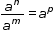must result in a whole-number value for p.
MA.7.NSO.1.2: Rewrite rational numbers in different but equivalent forms including fractions, mixed numbers, repeating decimals and percentages to solve mathematical and real-world problems.
 Examples:Justin is solving a problem where he computes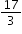and his calculator gives him the answer 5.6666666667. Justin makes the statement that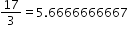; is he correct?
MA.8.AR.1.1: Apply the Laws of Exponents to generate equivalent algebraic expressions, limited to integer exponents and monomial bases.
 Clarifications:Clarification 1: Refer to the K-12 Formulas (Appendix E) for the Laws of Exponents.

 Examples:The expression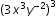is equivalent to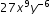.
MA.8.AR.1.2: Apply properties of operations to multiply two linear expressions with rational coefficients.
 Clarifications:Clarification 1: Problems are limited to products where at least one of the factors is a monomial. Clarification 2: Refer to Properties of Operations, Equality and Inequality (Appendix D).

 Examples:The product of (1.1+x) and (-2.3x) can be expressed as -2.53x-2.3x² or -2.3x²-2.53x.
MA.8.AR.1.3: Rewrite the sum of two algebraic expressions having a common monomial factor as a common factor multiplied by the sum of two algebraic expressions.
 Examples:The expression 99x-11x³ can be rewritten as 11x(9-x²) or as -11x(-9+x² ).
MA.8.AR.2.1: Solve multi-step linear equations in one variable, with rational number coefficients. Include equations with variables on both sides.
 Clarifications:Clarification 1: Problem types include examples of one-variable linear equations that generate one solution, infinitely many solutions or no solution.
MA.8.AR.2.2: Solve two-step linear inequalities in one variable and represent solutions algebraically and graphically.
 Clarifications:Clarification 1: Instruction includes inequalities in the forms px±q>r and p(x±q)>r, where p, q and r are specific rational numbers and where any inequality symbol can be represented. Clarification 2: Problems include inequalities where the variable may be on either side of the inequality.
MA.8.AR.2.3: Given an equation in the form of x²=p and x³=q, where p is a whole number and q is an integer, determine the real solutions.
 Clarifications:Clarification 1: Instruction focuses on understanding that when solving x²=p, there is both a positive and negative solution. Clarification 2: Within this benchmark, the expectation is to calculate square roots of perfect squares up to 225 and cube roots of perfect cubes from -125 to 125.
MA.8.AR.3.1: Determine if a linear relationship is also a proportional relationship.
 Clarifications:Clarification 1: Instruction focuses on the understanding that proportional relationships are linear relationships whose graph passes through the origin. Clarification 2: Instruction includes the representation of relationships using tables, graphs, equations and written descriptions.
MA.8.AR.3.2: Given a table, graph or written description of a linear relationship, determine the slope.
 Clarifications:Clarification 1: Problem types include cases where two points are given to determine the slope. Clarification 2: Instruction includes making connections of slope to the constant of proportionality and to similar triangles represented on the coordinate plane.
MA.8.AR.3.3: Given a table, graph or written description of a linear relationship, write an equation in slope-intercept form.
MA.8.AR.3.4: Given a mathematical or real-world context, graph a two-variable linear equation from a written description, a table or an equation in slope-intercept form.
MA.8.AR.3.5: Given a real-world context, determine and interpret the slope and y-intercept of a two-variable linear equation from a written description, a table, a graph or an equation in slope-intercept form.
 Clarifications:Clarification 1: Problems include conversions with temperature and equations of lines of fit in scatter plots.

 Examples:Raul bought a palm tree to plant at his house. He records the growth over many months and creates the equation h=0.21m+4.9, where h is the height of the palm tree in feet and m is the number of months. Interpret the slope and y-intercept from his equation.
MA.8.AR.4.1: Given a system of two linear equations and a specified set of possible solutions, determine which ordered pairs satisfy the system of linear equations.
 Clarifications:Clarification 1: Instruction focuses on the understanding that a solution to a system of equations satisfies both linear equations simultaneously.
MA.8.AR.4.2: Given a system of two linear equations represented graphically on the same coordinate plane, determine whether there is one solution, no solution or infinitely many solutions.
MA.8.AR.4.3: Given a mathematical or real-world context, solve systems of two linear equations by graphing.
 Clarifications:Clarification 1: Instruction includes approximating non-integer solutions. Clarification 2: Within this benchmark, it is the expectation to represent systems of linear equations in slope-intercept form only. Clarification 3: Instruction includes recognizing that parallel lines have the same slope.
MA.8.DP.1.1: Given a set of real-world bivariate numerical data, construct a scatter plot or a line graph as appropriate for the context.
 Clarifications:Clarification 1: Instruction includes recognizing similarities and differences between scatter plots and line graphs, and on determining which is more appropriate as a representation of the data based on the context. Clarification 2: Sets of data are limited to 20 points.

 Examples:Example: Jaylyn is collecting data about the relationship between grades in English and grades in mathematics. He represents the data using a scatter plot because he is interested if there is an association between the two variables without thinking of either one as an independent or dependent variable. Example: Samantha is collecting data on her weekly quiz grade in her social studies class. She represents the data using a line graph with time as the independent variable.
MA.8.DP.1.2: Given a scatter plot within a real-world context, describe patterns of association.
 Clarifications:Clarification 1: Descriptions include outliers; positive or negative association; linear or nonlinear association; strong or weak association.
MA.8.DP.1.3: Given a scatter plot with a linear association, informally fit a straight line.
 Clarifications:Clarification 1: Instruction focuses on the connection to linear functions. Clarification 2: Instruction includes using a variety of tools, including a ruler, to draw a line with approximately the same number of points above and below the line.
MA.8.DP.2.1: Determine the sample space for a repeated experiment.
 Clarifications:Clarification 1: Instruction includes recording sample spaces for repeated experiments using organized lists, tables or tree diagrams. Clarification 2: Experiments to be repeated are limited to tossing a fair coin, rolling a fair die, picking a card randomly from a deck with replacement, picking marbles randomly from a bag with replacement and spinning a fair spinner. Clarification 3: Repetition of experiments is limited to two times except for tossing a coin.
MA.8.DP.2.2: Find the theoretical probability of an event related to a repeated experiment.
 Clarifications:Clarification 1: Instruction includes representing probability as a fraction, percentage or decimal. Clarification 2: Experiments to be repeated are limited to tossing a fair coin, rolling a fair die, picking a card randomly from a deck with replacement, picking marbles randomly from a bag with replacement and spinning a fair spinner. Clarification 3: Repetition of experiments is limited to two times except for tossing a coin.
MA.8.DP.2.3: Solve real-world problems involving probabilities related to single or repeated experiments, including making predictions based on theoretical probability.
 Clarifications:Clarification 1: Instruction includes making connections to proportional relationships and representing probability as a fraction, percentage or decimal. Clarification 2: Experiments to be repeated are limited to tossing a fair coin, rolling a fair die, picking a card randomly from a deck with replacement, picking marbles randomly from a bag with replacement and spinning a fair spinner. Clarification 3: Repetition of experiments is limited to two times except for tossing a coin.

 Examples:Example: If Gabriella rolls a fair die 300 times, she can predict that she will roll a 3 approximately 50 times since the theoretical probability is. Example: Sandra performs an experiment where she flips a coin three times. She finds the theoretical probability of landing on exactly one head as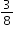. If she performs this experiment 50 times (for a total of 150 flips), predict the number of repetitions of the experiment that will result in exactly one of the three flips landing on heads.
MA.8.F.1.1: Given a set of ordered pairs, a table, a graph or mapping diagram, determine whether the relationship is a function. Identify the domain and range of the relation.
 Clarifications:Clarification 1: Instruction includes referring to the input as the independent variable and the output as the dependent variable. Clarification 2: Within this benchmark, it is the expectation to represent domain and range as a list of numbers or as an inequality.
MA.8.F.1.2: Given a function defined by a graph or an equation, determine whether the function is a linear function. Given an input-output table, determine whether it could represent a linear function.
 Clarifications:Clarification 1: Instruction includes recognizing that a table may not determine a function.
MA.8.F.1.3: Analyze a real-world written description or graphical representation of a functional relationship between two quantities and identify where the function is increasing, decreasing or constant.
 Clarifications:Clarification 1: Problem types are limited to continuous functions. Clarification 2: Analysis includes writing a description of a graphical representation or sketching a graph from a written description.
MA.8.GR.1.1: Apply the Pythagorean Theorem to solve mathematical and real-world problems involving unknown side lengths in right triangles.
 Clarifications:Clarification 1: Instruction includes exploring right triangles with natural-number side lengths to illustrate the Pythagorean Theorem. Clarification 2: Within this benchmark, the expectation is to memorize the Pythagorean Theorem. Clarification 3: Radicands are limited to whole numbers up to 225.
MA.8.GR.1.2: Apply the Pythagorean Theorem to solve mathematical and real-world problems involving the distance between two points in a coordinate plane.
 Clarifications:Clarification 1: Instruction includes making connections between distance on the coordinate plane and right triangles. Clarification 2: Within this benchmark, the expectation is to memorize the Pythagorean Theorem. It is not the expectation to use the distance formula. Clarification 3: Radicands are limited to whole numbers up to 225.

 Examples:The distance between (-2,7) and (0,6) can be found by creating a right triangle with the vertex of the right angle at the point (-2,6). This gives a height of the right triangle as 1 unit and a base of 2 units. Then using the Pythagorean Theorem the distance can be determined from the equation 1²+2²=c², which is equivalent to 5=c². So, the distance is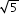units.
MA.8.GR.1.3: Use the Triangle Inequality Theorem to determine if a triangle can be formed from a given set of sides. Use the converse of the Pythagorean Theorem to determine if a right triangle can be formed from a given set of sides.
MA.8.GR.1.4: Solve mathematical problems involving the relationships between supplementary, complementary, vertical or adjacent angles.
MA.8.GR.1.5: Solve problems involving the relationships of interior and exterior angles of a triangle.
 Clarifications:Clarification 1: Problems include using the Triangle Sum Theorem and representing angle measures as algebraic expressions.
MA.8.GR.1.6: Develop and use formulas for the sums of the interior angles of regular polygons by decomposing them into triangles.
 Clarifications:Clarification 1: Problems include representing angle measures as algebraic expressions.
MA.8.GR.2.1: Given a preimage and image generated by a single transformation, identify the transformation that describes the relationship.
 Clarifications:Clarification 1: Within this benchmark, transformations are limited to reflections, translations or rotations of images. Clarification 2: Instruction focuses on the preservation of congruence so that a figure maps onto a copy of itself.
MA.8.GR.2.2: Given a preimage and image generated by a single dilation, identify the scale factor that describes the relationship.
 Clarifications:Clarification 1: Instruction includes the connection to scale drawings and proportions. Clarification 2: Instruction focuses on the preservation of similarity and the lack of preservation of congruence when a figure maps onto a scaled copy of itself, unless the scaling factor is 1.
MA.8.GR.2.3: Describe and apply the effect of a single transformation on two-dimensional figures using coordinates and the coordinate plane.
 Clarifications:Clarification 1: Within this benchmark, transformations are limited to reflections, translations, rotations or dilations of images.  Clarification 2: Lines of reflection are limited to the x-axis, y-axis or lines parallel to the axes. Clarification 3: Rotations must be about the origin and are limited to 90°, 180°, 270° or 360°. Clarification 4: Dilations must be centered at the origin.
MA.8.GR.2.4: Solve mathematical and real-world problems involving proportional relationships between similar triangles.
 Examples:During a Tampa Bay Lightning game one player, Johnson, passes the puck to his teammate, Stamkos, by bouncing the puck off the wall of the rink. The path of the puck creates two line segments that form hypotenuses for each of two similar right triangles, with the height of each triangle the distance from one of the players to the wall of the rink. If Johnson is 12 feet from the wall and Stamkos is 3 feet from the wall. How far did the puck travel from the wall of the rink to Stamkos if the distance traveled from Johnson to the wall was 16 feet?
MA.8.NSO.1.1: Extend previous understanding of rational numbers to define irrational numbers within the real number system. Locate an approximate value of a numerical expression involving irrational numbers on a number line.
 Clarifications:Clarification 1: Instruction includes the use of number line and rational number approximations, and recognizing pi (π) as an irrational number. Clarification 2: Within this benchmark, the expectation is to approximate numerical expressions involving one arithmetic operation and estimating square roots or pi (π).

 Examples:Within the expression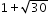, the irrational number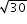can be estimated to be between 5 and 6 because 30 is between 25 and 36. By considering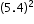and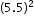, a closer approximation foris 5.5. So, the expressionis equivalent to about 6.5.
MA.8.NSO.1.2: Plot, order and compare rational and irrational numbers, represented in various forms.
 Clarifications:Clarification 1: Within this benchmark, it is not the expectation to work with the number e. Clarification 2: Within this benchmark, the expectation is to plot, order and compare square roots and cube roots. Clarification 3: Within this benchmark, the expectation is to use symbols (<, > or =).
MA.8.NSO.1.3: Extend previous understanding of the Laws of Exponents to include integer exponents. Apply the Laws of Exponents to evaluate numerical expressions and generate equivalent numerical expressions, limited to integer exponents and rational number bases, with procedural fluency.
 Clarifications:Clarification 1: Refer to the K-12 Formulas (Appendix E) for the Laws of Exponents.

 Examples:The expression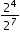is equivalent to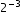which is equivalent to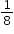.
MA.8.NSO.1.4: Express numbers in scientific notation to represent and approximate very large or very small quantities. Determine how many times larger or smaller one number is compared to a second number.
 Examples:Roderick is comparing two numbers shown in scientific notation on his calculator. The first number was displayed as 2.3147E27 and the second number was displayed as 3.5982E-5. Roderick determines that the first number is about 10³² times bigger than the second number.
MA.8.NSO.1.5: Add, subtract, multiply and divide numbers expressed in scientific notation with procedural fluency.
 Clarifications:Clarification 1: Within this benchmark, for addition and subtraction with numbers expressed in scientific notation, exponents are limited to within 2 of each other.

 Examples:The sum of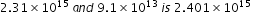.
MA.8.NSO.1.6: Solve real-world problems involving operations with numbers expressed in scientific notation.
 Clarifications:Clarification 1: Instruction includes recognizing the importance of significant digits when physical measurements are involved. Clarification 2: Within this benchmark, for addition and subtraction with numbers expressed in scientific notation, exponents are limited to within 2 of each other.
MA.8.NSO.1.7: Solve multi-step mathematical and real-world problems involving the order of operations with rational numbers including exponents and radicals.
 Clarifications:Clarification 1: Multi-step expressions are limited to 6 or fewer steps. Clarification 2: Within this benchmark, the expectation is to simplify radicals by factoring square roots of perfect squares up to 225 and cube roots of perfect cubes from -125 to 125.

 Examples:The expression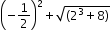is equivalent to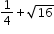which is equivalent to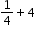which is equivalent to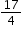.
MA.K12.MTR.1.1: Actively participate in effortful learning both individually and collectively.

Mathematicians who participate in effortful learning both individually and with others:

• Analyze the problem in a way that makes sense given the task.
• Build perseverance by modifying methods as needed while solving a challenging task.
• Stay engaged and maintain a positive mindset when working to solve tasks.
• Help and support each other when attempting a new method or approach.

 Clarifications:Teachers who encourage students to participate actively in effortful learning both individually and with others: Cultivate a community of growth mindset learners.  Foster perseverance in students by choosing tasks that are challenging. Develop students’ ability to analyze and problem solve. Recognize students’ effort when solving challenging problems.
MA.K12.MTR.2.1: Demonstrate understanding by representing problems in multiple ways.

Mathematicians who demonstrate understanding by representing problems in multiple ways:

• Build understanding through modeling and using manipulatives.
• Represent solutions to problems in multiple ways using objects, drawings, tables, graphs and equations.
• Progress from modeling problems with objects and drawings to using algorithms and equations.
• Express connections between concepts and representations.
• Choose a representation based on the given context or purpose.
 Clarifications:Teachers who encourage students to demonstrate understanding by representing problems in multiple ways: Help students make connections between concepts and representations.Provide opportunities for students to use manipulatives when investigating concepts.Guide students from concrete to pictorial to abstract representations as understanding progresses.Show students that various representations can have different purposes and can be useful in different situations.
MA.K12.MTR.3.1: Complete tasks with mathematical fluency.

Mathematicians who complete tasks with mathematical fluency:

• Select efficient and appropriate methods for solving problems within the given context.
• Maintain flexibility and accuracy while performing procedures and mental calculations.
• Complete tasks accurately and with confidence.
• Adapt procedures to apply them to a new context.
• Use feedback to improve efficiency when performing calculations.
 Clarifications:Teachers who encourage students to complete tasks with mathematical fluency:Provide students with the flexibility to solve problems by selecting a procedure that allows them to solve efficiently and accurately.Offer multiple opportunities for students to practice efficient and generalizable methods.Provide opportunities for students to reflect on the method they used and determine if a more efficient method could have been used.
MA.K12.MTR.4.1: Engage in discussions that reflect on the mathematical thinking of self and others.

Mathematicians who engage in discussions that reflect on the mathematical thinking of self and others:

• Communicate mathematical ideas, vocabulary and methods effectively.
• Analyze the mathematical thinking of others.
• Compare the efficiency of a method to those expressed by others.
• Recognize errors and suggest how to correctly solve the task.
• Justify results by explaining methods and processes.
• Construct possible arguments based on evidence.
 Clarifications:Teachers who encourage students to engage in discussions that reflect on the mathematical thinking of self and others:Establish a culture in which students ask questions of the teacher and their peers, and error is an opportunity for learning.Create opportunities for students to discuss their thinking with peers.Select, sequence and present student work to advance and deepen understanding of correct and increasingly efficient methods.Develop students’ ability to justify methods and compare their responses to the responses of their peers.
MA.K12.MTR.5.1: Use patterns and structure to help understand and connect mathematical concepts.

Mathematicians who use patterns and structure to help understand and connect mathematical concepts:

• Focus on relevant details within a problem.
• Create plans and procedures to logically order events, steps or ideas to solve problems.
• Decompose a complex problem into manageable parts.
• Relate previously learned concepts to new concepts.
• Look for similarities among problems.
• Connect solutions of problems to more complicated large-scale situations.
 Clarifications:Teachers who encourage students to use patterns and structure to help understand and connect mathematical concepts:Help students recognize the patterns in the world around them and connect these patterns to mathematical concepts.Support students to develop generalizations based on the similarities found among problems.Provide opportunities for students to create plans and procedures to solve problems.Develop students’ ability to construct relationships between their current understanding and more sophisticated ways of thinking.
MA.K12.MTR.6.1: Assess the reasonableness of solutions.

Mathematicians who assess the reasonableness of solutions:

• Estimate to discover possible solutions.
• Use benchmark quantities to determine if a solution makes sense.
• Check calculations when solving problems.
• Verify possible solutions by explaining the methods used.
• Evaluate results based on the given context.
 Clarifications:Teachers who encourage students to assess the reasonableness of solutions:Have students estimate or predict solutions prior to solving.Prompt students to continually ask, “Does this solution make sense? How do you know?”Reinforce that students check their work as they progress within and after a task.Strengthen students’ ability to verify solutions through justifications.
MA.K12.MTR.7.1: Apply mathematics to real-world contexts.

Mathematicians who apply mathematics to real-world contexts:

• Connect mathematical concepts to everyday experiences.
• Use models and methods to understand, represent and solve problems.
• Perform investigations to gather data or determine if a method is appropriate. • Redesign models and methods to improve accuracy or efficiency.
 Clarifications:Teachers who encourage students to apply mathematics to real-world contexts:Provide opportunities for students to create models, both concrete and abstract, and perform investigations.Challenge students to question the accuracy of their models and methods.Support students as they validate conclusions by comparing them to the given situation.Indicate how various concepts can be applied to other disciplines.
ELA.K12.EE.1.1: Cite evidence to explain and justify reasoning.
 Clarifications:K-1 Students include textual evidence in their oral communication with guidance and support from adults. The evidence can consist of details from the text without naming the text. During 1st grade, students learn how to incorporate the evidence in their writing.2-3 Students include relevant textual evidence in their written and oral communication. Students should name the text when they refer to it. In 3rd grade, students should use a combination of direct and indirect citations.4-5 Students continue with previous skills and reference comments made by speakers and peers. Students cite texts that they’ve directly quoted, paraphrased, or used for information. When writing, students will use the form of citation dictated by the instructor or the style guide referenced by the instructor. 6-8 Students continue with previous skills and use a style guide to create a proper citation.9-12 Students continue with previous skills and should be aware of existing style guides and the ways in which they differ.
 Clarifications:See Text Complexity for grade-level complexity bands and a text complexity rubric.
ELA.K12.EE.3.1: Make inferences to support comprehension.
 Clarifications:Students will make inferences before the words infer or inference are introduced. Kindergarten students will answer questions like “Why is the girl smiling?” or make predictions about what will happen based on the title page. Students will use the terms and apply them in 2nd grade and beyond.
ELA.K12.EE.4.1: Use appropriate collaborative techniques and active listening skills when engaging in discussions in a variety of situations.
 Clarifications:In kindergarten, students learn to listen to one another respectfully.In grades 1-2, students build upon these skills by justifying what they are thinking. For example: “I think ________ because _______.” The collaborative conversations are becoming academic conversations.In grades 3-12, students engage in academic conversations discussing claims and justifying their reasoning, refining and applying skills. Students build on ideas, propel the conversation, and support claims and counterclaims with evidence.
ELA.K12.EE.5.1: Use the accepted rules governing a specific format to create quality work.
 Clarifications:Students will incorporate skills learned into work products to produce quality work. For students to incorporate these skills appropriately, they must receive instruction. A 3rd grade student creating a poster board display must have instruction in how to effectively present information to do quality work.
ELA.K12.EE.6.1: Use appropriate voice and tone when speaking or writing.
 Clarifications:In kindergarten and 1st grade, students learn the difference between formal and informal language. For example, the way we talk to our friends differs from the way we speak to adults. In 2nd grade and beyond, students practice appropriate social and academic language to discuss texts.
ELD.K12.ELL.MA.1: English language learners communicate information, ideas and concepts necessary for academic success in the content area of Mathematics.

## General Course Information and Notes

### VERSION DESCRIPTION

In grade 7 accelerated, instructional time will emphasize six areas: (1) representing numbers in scientific notation and extending the set of numbers to the system of real numbers, which includes irrational numbers; (2) generating equivalent numeric and algebraic expressions including using the Laws of Exponents; (3) creating and reasoning about linear relationships including modeling an association in bivariate data with a linear equation; (4) solving linear equations, inequalities and systems of linear equations; (5) developing an understanding of the concept of a function and (6) analyzing two-dimensional figures, particularly triangles, using distance, angle and applying the Pythagorean Theorem.

Curricular content for all subjects must integrate critical-thinking, problem-solving, and workforce-literacy skills; communication, reading, and writing skills; mathematics skills; collaboration skills; contextual and applied-learning skills; technology-literacy skills; information and media-literacy skills; and civic-engagement skills.

### GENERAL NOTES

Honors and Accelerated Level Course Note: Accelerated courses require a greater demand on students through increased academic rigor.  Academic rigor is obtained through the application, analysis, evaluation, and creation of complex ideas that are often abstract and multi-faceted.  Students are challenged to think and collaborate critically on the content they are learning. Honors level rigor will be achieved by increasing text complexity through text selection, focus on high-level qualitative measures, and complexity of task. Instruction will be structured to give students a deeper understanding of conceptual themes and organization within and across disciplines. Academic rigor is more than simply assigning to students a greater quantity of work.

Florida’s Benchmarks for Excellent Student Thinking (B.E.S.T.) Standards
This course includes Florida’s B.E.S.T. ELA Expectations (EE) and Mathematical Thinking and Reasoning Standards (MTRs) for students. Florida educators should intentionally embed these standards within the content and their instruction as applicable. For guidance on the implementation of the EEs and MTRs, please visit https://www.cpalms.org/Standards/BEST_Standards.aspx and select the appropriate B.E.S.T. Standards package.

English Language Development ELD Standards Special Notes Section:
Teachers are required to provide listening, speaking, reading and writing instruction that allows English language learners (ELL) to communicate information, ideas and concepts for academic success in the content area of Mathematics. For the given level of English language proficiency and with visual, graphic, or interactive support, students will interact with grade level words, expressions, sentences and discourse to process or produce language necessary for academic success. The ELD standard should specify a relevant content area concept or topic of study chosen by curriculum developers and teachers which maximizes an ELL’s need for communication and social skills. To access an ELL supporting document which delineates performance definitions and descriptors, please click on the following link:

### General Information

 Course Number: 1205050 Course Path: Section: Grades PreK to 12 Education Courses > Grade Group: Grades 6 to 8 Education Courses > Subject: Mathematics > SubSubject: General Mathematics > Abbreviated Title: M/J ACCEL MATH GR 7 Course Attributes: Honors Class Size Core Required Florida Standards Course Course Type: Core Academic Course Course Level: 3 Course Status: State Board Approved Grade Level(s): 7

#### Educator Certifications

 Carnegie Learning FL Middle School Math Solution, Grade 7 Accelerated MathematicsSandy Bartle Finocchi and Amy Jones Lewis - Carnegie Learning, Inc. dba EMC Publishing & Mondo Ed - 1st - 2023enVision Florida B.E.S.T. Mathematics Grade 7 AcceleratedBerry, et al - Savvas Learning Company LLC, formerly known as Pearson K12 Learning LLC. - 1 - 2023Florida EdGems Math Course 2 AcceleratedShannon McCaw - EdGems Math LLC - 1st - 2022Florida Reveal Math, Grade 7 AcceleratedCathy L. Seeley , Ed.D; Raj Shah, Ph.D.; Cheryl R. Tobey, M.Ed.; Dinah Zike, M.Ed.; Walter Secada, Ph.D. - McGraw Hill LLC - 1 - 2023Florida's B.E.S.T. Standards for MATH Grade 7 Accelerated with CalcChat® and CalcView®Ron Larson and Laurie Boswell - Big Ideas Learning, LLC - 1 - 2023HMH Florida's B.E.S.T. Into Math Accelerated 7Edward B. Burger, PhD; Juli K. Dixon, PhD; Timothy D. Kanold, PhD; Matthew R. Larson, PhD; Steven J. Leinwand, PhD - Houghton Mifflin Harcourt - N/A - 2023Math Nation: Florida's B.E.S.T. 7th Grade Accelerated MathMath Nation - Math Nation (a division of Study Edge) - 1 - 2023